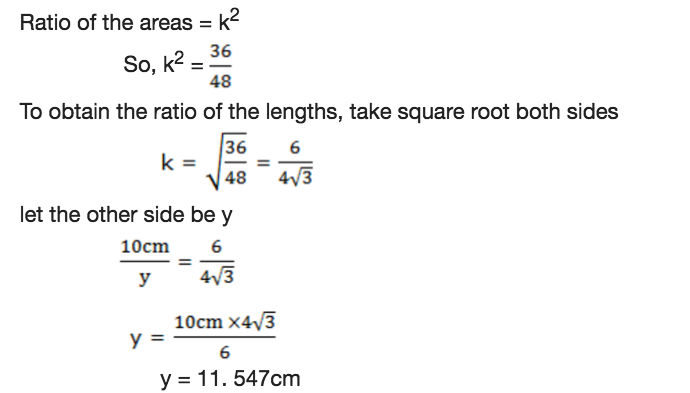error: Content is protected !!

# MATHEMATICS: FORM FOUR: Topic 2 - AREA AND PERIMETER

TOPIC 2: AREA AND PERIMETER

The Formula for the Area of any Triangle
Derive the formula for the area of any triangle
Area of triangle is given by½bh, whereby b is the base of the triangle and h is the height of the given triangle. Consider the illustrations below: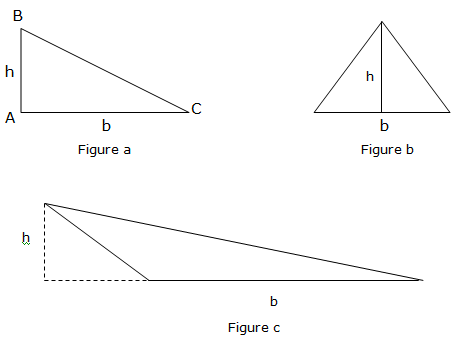From the figure above, we see where the base and height are located.
Applying the Formula to find the Area of any Triangle
Apply the formula to find the area of any triangle
Example 1
The base of a triangle is 12cm long. If the corresponding height is 7cm, find the area of the triangle.
Solution
Consider the figure below: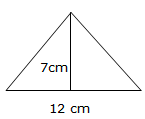The area of a triangle is given by½bh.
Area =½×12cm×7cm
Area = 42cm2
Therefore area of a triangle is 42cm2
Example 2
The lengths of two sides of a triangle are 6cm and 8cm. Find the area of a triangle if the included is
Solution:
Consider the triangle below, name it triangle ABC.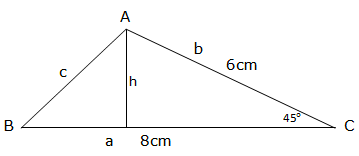The area of a triangle above is given by½b×h
So, Area = ½× 8cm× 6cm × sin 45°
=24cm2× sin45°
= 16.97cm2
Therefore the area of ABC = 16.97cm2
The Formula for Finding the Area of Rhombi in Terms of the Diagonals
Derive the formula for finding the area of rhombi in terms of the diagonals.
The area of a rhombus is the same as the area of a parallelogram because rhombus is a special kind of parallelogram. Rhombus is a parallelogram with equal sides. Consider the figure below of a rhombus with base b and height h.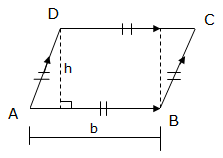So, Area of rhombus = bh
Another formula for finding the area of rhombus can be obtained by using the diagonals. Consider the rhombus below: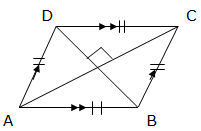Diagonals of a rhombus bisect each other at right angles (means the diagonal lines are half equally), so the area of a rhombus ABCD can be found as follows:
Area of a triangle ABC = area of a triangle ADC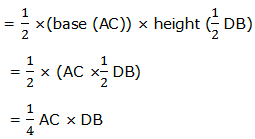Since the triangles are equal, the area of ABCD: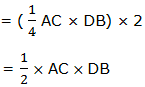Therefore, the area of a rhombus is equal to half the product of the length of the diagonals.

The Formula to Find the Areas of Quadrilaterals
Apply the formula to find the areas of quadrilaterals
Area of a Trapezium
Consider the trapezium with constructed lines as shown in the figure below: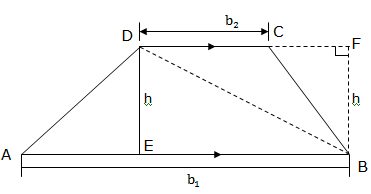In order to find the area of a trapezium, first let us find the area of the triangles ABD and BDC with the same height h.
The base of the triangle ABD is b1
The base of the triangle BDC is b2
The area of the triangle ABD = ½b1h
The area of the triangle BDC = ½b2h
Area of ABDC =½b1h +½b2h
Area of ABDC=½ (b1 + b2)h
Generally, the area of the trapezium is given by½ (b1+ b2)hor is the product of half the sum of the parallel sides (bases) and the perpendicular distance between them (height)
Example 3
Find the height of the trapezium with area 90 square units and bases of 6 units and 14 units
Solution:
Consider the trapezium below: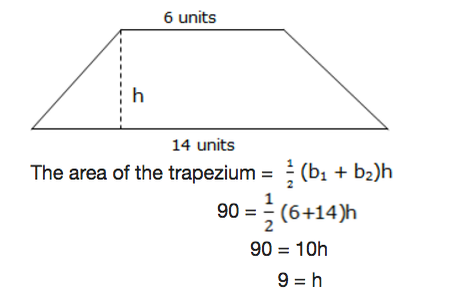Therefore the height of the trapezium is 9 units.
Area of a Parallelogram
Consider parallelogram below with constructed lines as shown in the figure.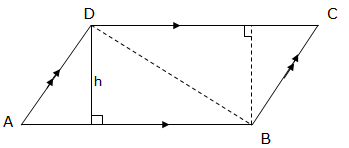The area of the parallelogram can be formed from the formula for the area of the trapezium. The important thing to note is that the bases for a parallelogram are equal.
Now, the area of the parallelogram ABCD=½ (AB+DC)hsquare units.Since AB = DC
Then, the area of parallelogram ABCD =½ × 2AB × h = AB× h
If AB =b, then Area of the parallelogram ABCD = bh, where b is the base and h is the height of a parallelogram.
Therefore; the area of a parallelogram is equal to the product of the base and the perpendicular height.
Area of a Rectangle
Consider the rectangle below: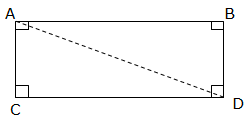The rectangle ABCD is divided into two congruent triangles, which are triangle ABD and triangle ACD by the diagonal AD.
The area of ABCD = area of triangle ABD + area of triangle ACD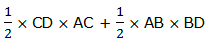Since the triangles are equal, the area of ABCD is equals to double the area of one of the triangles.The area of ABCD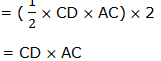If CD is the length 'l'of the Rectangle and AC is the width 'w' of the Rectangle, then, the area of ABCD = l×or lw
Therefore, the area of the Rectangle is the product of the length and width.
Area of a Square
A Square is a special rectangle with equal sides. Therefore the area of the square is the product of its lengths. i.e. Area of a square = l × l=l2.
We can also find the area of a square by using the length of the diagonals. Consider the square below with diagonals AC and DB: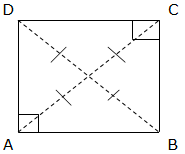Each of the diagonals of a square bisect at a right angle.Area of a triangle ABC = Area of ADC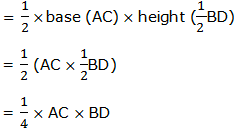But the area of ABCD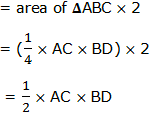Since the length of the diagonals are equal, then AC = BD.So, the area of ABCD = ½ (AC)2
Therefore the area of a Square is equals to the half of the product of the lengths of the diagonals.
Example 4
Find the area of a parallelogram ABCD if AC = 7cm, AB = 9cm and the angle ZWX =.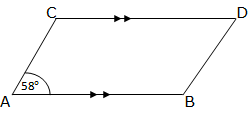Solution
Area of a parallelogram = base×height
Consider the figure below: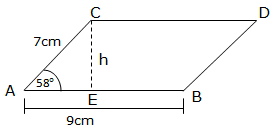Consider the triangle ACE to find h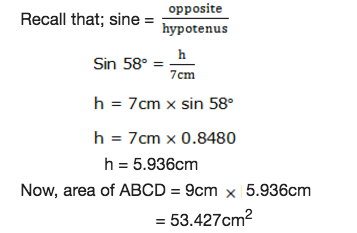Therefore the area of the parallelogram ABCD is 53.427cm2.

The Formula for Finding the Length of a Side of a Regular Polygon
Derive the formula for finding the length of a side of a regular polygon
When we sum up the lengths of the sides of the polygon we obtain what is called perimeter of a polygon. Therefore, perimeter of a regular polygon is the sum of the lengths of the sides of the polygon.
How to find the perimeter of a Regular Polygon inscribed in a circle?
An inscribed polygon is the one whose vertices lie on the circle. If the lengths of the sides of the polygon are the same we say that the polygon is an inscribed Regular Polygon.
A Regular Polygon with number of sides larger than 2 say n sides can be inscribed in a circle as follows:
For example, if you want to construct an inscribed regular hexagon (6 sides), first draw a circle and locate the center of the circle. Then draw rays that intersect the circle in six points from the center of the circle. Each angle at the center will measure 360°/6 = 60°. Connect the points of intersection on the circle by line segments. The figure formed is an inscribed regular polygon. See the figure below: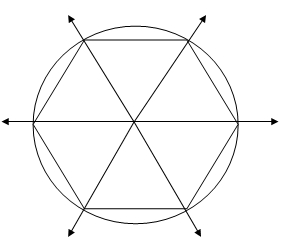Now, to obtain the formula of finding the perimeter of a regular polygon inscribed in a circle with radius r and center O, let AB be the side of the polygon and OC the perpendicular from O to AB as shown in the figure below: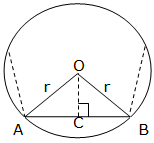The angle AOB = 360°/n, since the polygon has n sides.
The angle AOC = the angle AOB and the angle BOC = angle AOC +angle BOC
Therefore, the angle AOC = ½(360°/n) = 180°/n
Let the length of the side of the regular polygon ABbe S.
Then,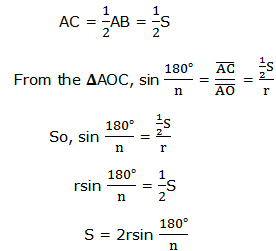Therefore the length of a regular polygon with n sides inscribed in a circle is given by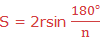The Perimeter of a Regular Polygon
The formula to determine the perimeter of a regular polygon
If we let 2r = d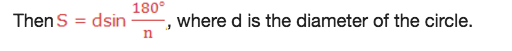From the concept of perimeter that perimeter of a regular polygon is the sum of the lengths of the sides of the polygon , if we have n sides each with length ‘S’ then the sum of the lengths of these sides will be nS. Therefore, Perimeter P of a regular polygon of n sides each with length S is given by: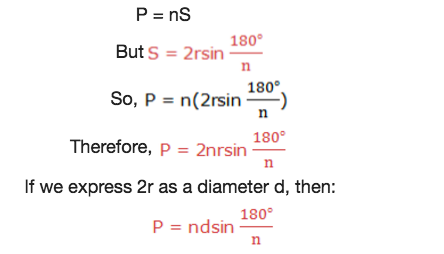Example 5
Find the length of one side of eight-sided regular polygon inscribed in a circle with radius 7cm.
Solution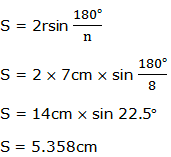Therefore the length of one side of eight-sided regular polygon with radius of 7cm is 5.358cm

The Formula for Finding the Area of a Regular Polygon
Derive the formula for finding the area of a regular polygon
Consider the regular polygon with n sides inscribed in a circle of radius r and center O as shown below: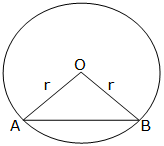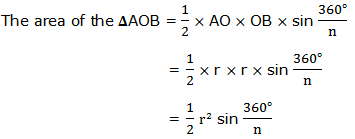But since each vertex of the polygon is connected to O, the polygon region is divided into n triangles which are equal.
Now,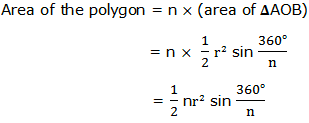Therefore the area of a polygon of n sides inscribed in a circle of radius r is given by: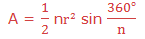The Formula to Calculate the Area of a Regular Polygon
Apply the formula to calculate the area of a regular polygon
Example 6
Find the area of twelve-sided regular polygon inscribed in a circle of radius 14 cm
Solution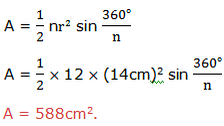Circumference and area of a circle
Circumference of a circle is the distance around it. Circumference of a circle can be estimated by using a regular polygon with many sides inscribed in a circle with radius r.
We know perimeter of the regular polygon is given by: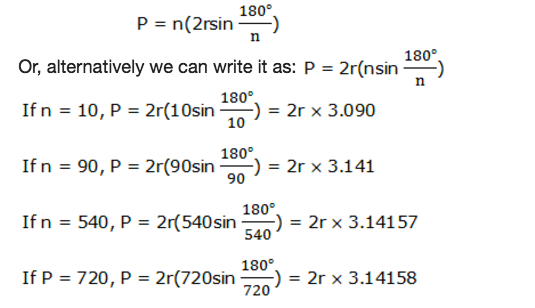Here we see that, as n increases the value of nsin 180°/napproaches the value ofπ.When n is very large the perimeter of a regular polygon approaches the circumference of the circle. The value ofnsin 180°/ncan be replaced byπbecause it approaches the value ofπwhen n is very large.
Therefore, circumference of the circle C, is given by C = 2πr
Area of a Circle
In similar way we can generate the formula of calculating the area of a circle by considering area of a regular polygon inscribed in a circle of radius r.
We know that, area of a regular polygon is given by: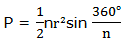Alternatively we can write it as: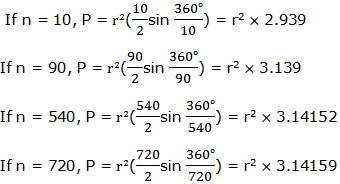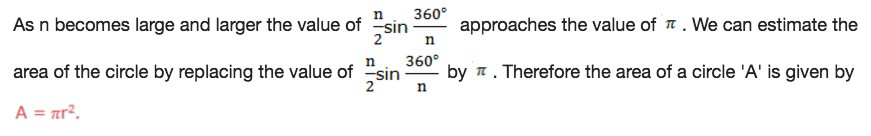Example 7
Find the circumference of a circle of radius 21cm. (takeπ= 3.14).
Solution
Circumference of a circle, C = 2πr
= 2× 3.14 × 21cm = 131.88cm

The Ratio of Areas of Similar Polygons
Find the ratio of areas of similar polygons
Let ABC and A’ B’ C’ be two similar triangles: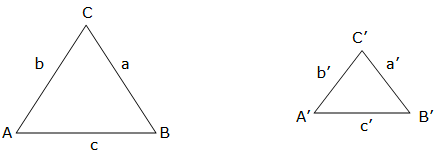If we find the ratio of their sides we get;Generally, if the ratio of the lengths of the corresponding sides of two similar polygons is k, then the ratio of their areas is k2.
Problems Related to Ratio for Areas of Similar Polygons
Solve problems related to ratio for areas of similar polygons
Example 8
We are given two triangles which are similar. The length of one side is 8cm and the length of the corresponding side is 14cm. if the area of a smaller triangle is 24cm2find the area of the other triangle.
Solution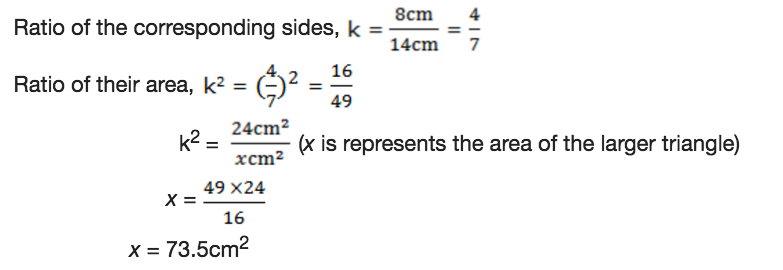Therefore the area of the other triangle is 73.5cm2.
Example 9
The ratio of the areas of two similar polygons is 36:48. The length of a side of the smaller polygon is 10cm. find the length of the corresponding side of the other polygon.
Solution